###### back to index | new

In $\triangle BAC$, $\angle BAC=40^\circ$, $AB=10$, and $AC=6$. Points $D$ and $E$ lie on $\overline{AB}$ and $\overline{AC}$ respectively. What is the minimum possible value of $BE+DE+CD$?

Suppose the point $F$ is inside a square $ABCD$ such that $BF=1$, $FA=2$, and $FD=3$, as shown. Find the measurement of $\angle{BFA}$.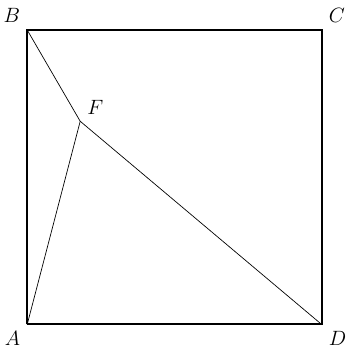In $\triangle{ABC}$, if $\sin{A}:\sin{B}:\sin{C}=4:5:7$, compute $\cos{C}$ and $\sin{C}$.

In $\triangle{ABC}$, if $(a+b+c)(a+b-c)=3ab$, compute $\angle{C}$.

Two people located 500 yards apart have spotted a hot air balloon. The angle of elevation from one person to the balloon is $67^\circ$. From the second person to the balloon the angle of elevation is $46^\circ$. How high is the balloon when it is spotted? (You can use a calculator. Please keep the result to the 2 decimal places.)

In $\triangle{ABC}$, if $(a^2 +b^2)\sin(A-B)=(a^2-b^2)\sin(A+B)$, determine the shape of $\triangle{ABC}$.

Let $\triangle{ABC}$ be an acute triangle and $a, b, c$ be the three sides opposite to $\angle{A}, \angle{B}, \angle{C}$ respectively. If vectors $m=(a+c,b)$ and $n=(a-c, b-a)$ satisfy $m\cdot n = 0$, (1) Compute the measurement of $\angle{C}$. (2) Find the range of $\sin{A} + \sin{B}$.

In $\triangle{ABC}$, if $a\cos{C} + \frac{c}{2} = b$, (1) compute $\angle{A}$. (2) if $a=1$, find the range of the perimeter o f $\triangle{ABC}$.

Let $BD$ be a median in $\triangle{ABC}$. If $AB=\frac{4\sqrt{6}}{3}$, $\cos{B}=\dfrac{\sqrt{6}}{6}$, and $BD=\sqrt{5}$, find the length of $BC$ and the value of $\sin{A}$.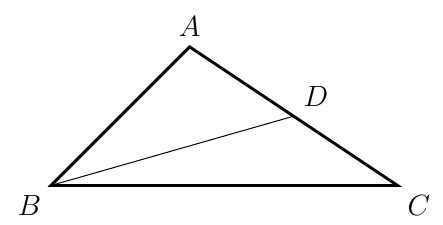Let $ABCD$ be inscribed in a circle. If $AB=a, BC=b, CD=c,$ and $DA=d$, show that $$\cos{B} = \frac{a^2 + b^2 -c^2 - d^2}{2(ab+cd)}$$

There are four points on a plane as shown. Points $A$ and $B$ are fixed points satisfying $AB=\sqrt{3}$. Points $P$ and $Q$ can move, as long as $AP=PQ=QB=1$. Let $S$ and $T$ be the area of $\triangle{APB}$ and $\triangle{PQB}$, respectively. Find the maximum value of $S^2+T^2$.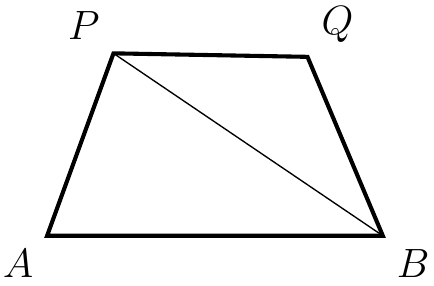Let $R=\frac{7\sqrt{3}}{3}$ be the circumradius of $\triangle{ABC}$. If $\angle{B} = 60^\circ$ and its area $S_{\triangle{ABC}}=10\sqrt{3}$, find the lengths of $a$, $b$, and $c$.

In $\triangle{ABC}$, $AB = 33, AC=21,$ and $BC=m$ where $m$ is an integer. There exist points $D$ and $E$ on $AB$ and $AC$, respectively, such that $AD=DE=EC=n$ where $n$ is also an integer. Find all the possible values of $m$.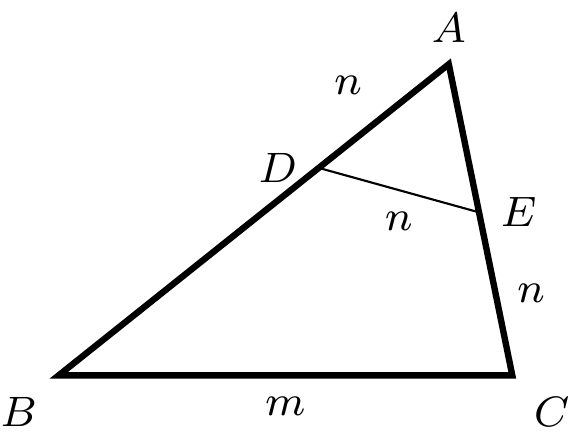Let quadrilateral $ABCD$ inscribe a circle. If $BE=ED$, prove $$AB^2+BC^2 +CD^2 + DA^2 = 2AC^2$$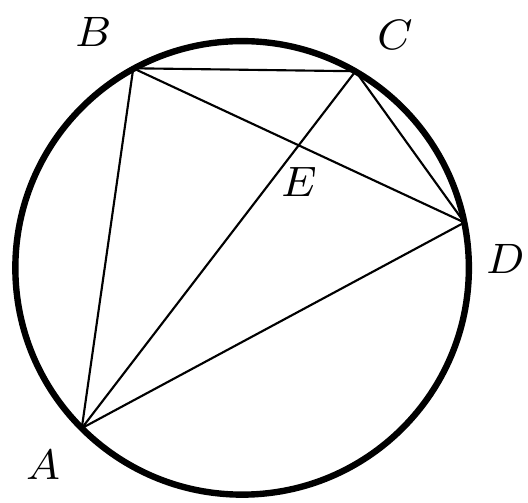In $\triangle{ABC}$, $AE$ and $AF$ trisects $\angle{A}$, $BF$ and $BD$ trisects $\angle{B}$, $CD$ and $CE$ trisects $\angle{C}$. Show that $\triangle{DEF}$ is equilateral.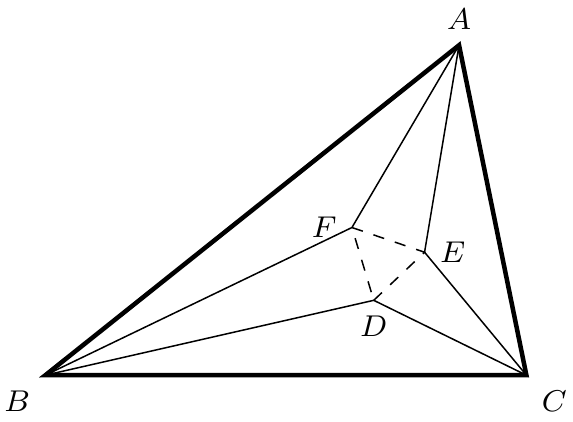In tetrahedron $ABCD$, $\angle{ADB} = \angle{BDC} = \angle{CDA} = 60^\circ$, $AD=BD=3$, and $CD=2$. Find the radius of $ABCD$'s circumsphere.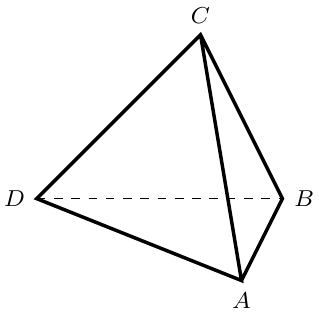Show that if $a, b, c$ are the lengths of the sides of a triangle, then the equation $$b^2x^2 +(b^2+c^2-a^2)x+c^2=0$$ does not have real roots.

Given two segments $AB$ and $MN$, show that $$MN\perp AB \Leftrightarrow AM^2 - BM^2 = AN^2 - BN^2$$

In $\triangle{ABC}$, let $a$, $b$, and $c$ be the lengths of sides opposite to $\angle{A}$, $\angle{B}$ and $\angle{C}$, respectively. $D$ is a point on side $AB$ satisfying $BC=DC$. If $AD=d$, show that $$c+d=2\cdot b\cdot\cos{A}\quad\text{and}\quad c\cdot d = b^2-a^2$$

As shown, both $ABCD$ and $OPRQ$ are squares. Additionally, $O$ is the center of $ABCD$, $OP=1$, $BP=\sqrt{2}$, and $CQ=\sqrt{5}$. Find the length of $DR$.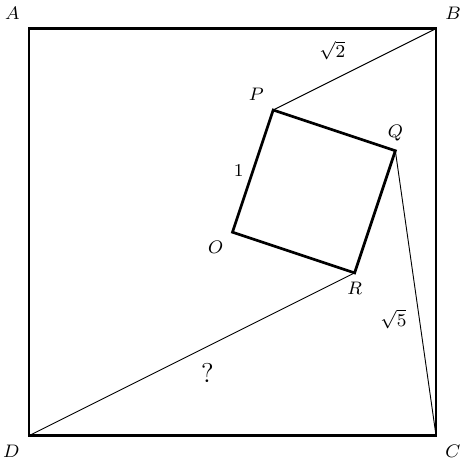In $\triangle ABC, AB = AC = 10$ and $BC = 12$. Point $D$ lies strictly between $A$ and $B$ on $\overline{AB}$ and point $E$ lies strictly between $A$ and $C$ on $\overline{AC}$ so that $AD = DE = EC$. Then $AD$ can be expressed in the form $\dfrac{p}{q}$, where $p$ and $q$ are relatively prime positive integers. Find $p+q$.

Regular octagon $ABCDEFGH$ has area $n$. Let $m$ be the area of quadrilateral $ACEG$. What is $\tfrac{m}{n}?$